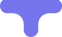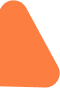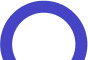>### Master Math with 1:1 Online Classes. Learn from Experts!# Prime Numbers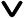• Exploring Prime Numbers: Unlocking the Beauty of Mathematics
• What are Prime Numbers?
• Understanding Prime Numbers with Visual Models
• The Significance of Prime Numbers
• The History of Prime Numbers
• The Sieve of Eratosthenes
• Prime Numbers Chart 1 to 100
• Twin Prime Numbers
• Difference between Co-Prime Numbers and Prime Numbers
• Interesting Facts about Prime Numbers
• Solved Examples
• Practice Problems

## Exploring Prime Numbers: Unlocking the Beauty of Mathematics

Numbers are incredibly fascinating, with numerous ways to interpret and analyze them. Let's delve into the captivating realm of prime numbers and uncover the secrets they hold.

## What are Prime Numbers?

Prime numbers are special numbers that possess unique properties in mathematics. They have only two factors: 1 and the number itself. For example, the numbers 5 and 7 are prime numbers, as they can only be divided evenly by 1 and themselves.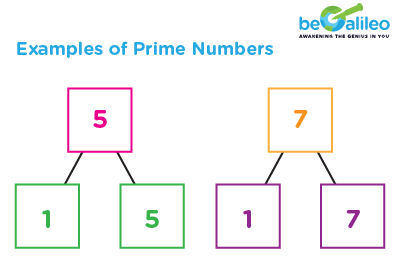Prime numbers are the building blocks of the number system, playing crucial role in various mathematical concepts and calculations. They have fascinated mathematicians for centuries due to their elusive nature and intriguing patterns.

## Understanding Prime Numbers with Visual Models

The concept of prime numbers is fascinating and can be explored through simple visualizations. Let's consider a scenario with marbles to understand it better.Imagine you have 5 marbles. How many different ways can you arrange them in equal groups?

By carefully examining the marbles, you'll notice that there are only two ways to arrange them: you can either keep all 5 marbles together as a single group or separate them into individual groups of 1 marble each.Now, let's consider another scenario with 7 marbles. Similarly, in how many ways can you arrange them in equal groups?

Upon observation, you'll find that again there are only two ways: keeping all 7 marbles together or arranging them into individual groups of 1 marble each.

Through these simple examples, we can observe that certain numbers have a unique property of having exactly two factors: 1 and the number itself. In other words, prime numbers are not divisible by any other number except 1 and itself. For instance, the numbers 2, 3, 5, and 7 are prime numbers because they satisfy this criterion.

## The Significance of Prime Numbers

Prime numbers hold a significant place in the world of mathematics. They are the fundamental building blocks from which other numbers emerge through multiplication.

Understanding prime numbers helps us comprehend the structure and patterns within the realm of numbers. Prime numbers find extensive applications in number theory, cryptography, and algorithms. Their unique properties make them essential in modern encryption methods used to protect sensitive information from cyber-crimes.

## The History of Prime Numbers

The exploration of prime numbers dates back to ancient times. Greek mathematician Euclid mentioned prime numbers in his work 'Elements,' recognizing their importance and studying their properties. However, it was another Greek mathematician, Eratosthenes, who made significant contributions to understanding prime numbers.

Eratosthenes invented the renowned "Sieve of Eratosthenes" algorithm, which aids in identifying prime numbers. This method, developed over two thousand years ago, is still widely used today.

However, it was not until the 17th century that mathematicians like Fermat, Euler, and Gauss began to examine the structure within prime numbers.

## The Sieve of Eratosthenes

Let's explore the Sieve of Eratosthenes, a method developed by Eratosthenes, to identify prime numbers between 1 and 100.

The Sieve of Eratosthenes works by systematically crossing out composite numbers, leaving only the prime numbers behind. Here's how it works:

Step 1: Begin by listing all numbers from 1 to 100.Step 2: Start with the first prime number, which is 2. Circle it and cross out all multiples of 2, excluding 2 itself.Step 3: Move to the next available number, which is 3. Circle it and cross out all multiples of 3, excluding 3 itself.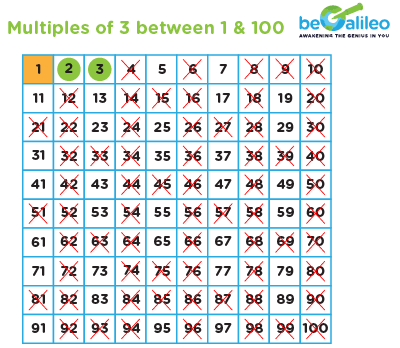Step 4: Repeat the process with the remaining prime numbers (5, 7, 11, etc.), circling them and crossing out their multiples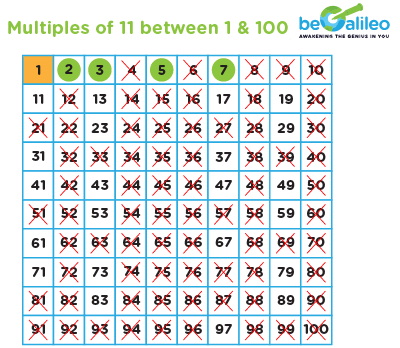Step 5: Continue this process until you reach the end of the number grid.The circled numbers that remain are the prime numbers between 1 and 100, as determined by the Sieve of Eratosthenes.

## Prime Numbers Chart 1 to 100

A prime numbers chart systematically represents all prime numbers from 1 to 100. Prime numbers, except for 2, are odd numbers. The chart below lists all the prime numbers from 1 to 100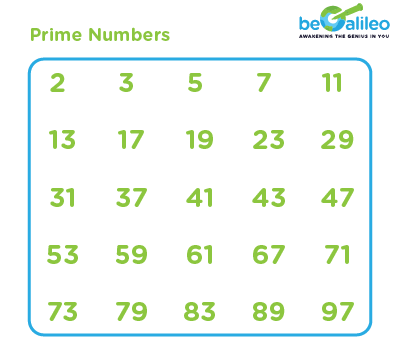## Twin Prime Numbers

Twin prime numbers are a fascinating and intriguing concept within the realm of prime numbers. Twin primes are pairs of prime numbers that differ by 2. In other words, they are prime numbers that have a difference of only 2 between them. For example, (3, 5), (5, 7), (11, 13), and (17, 19) are all examples of twin prime pairs.## Difference between Co-Prime Numbers and Prime Numbers

Co-prime numbers, also known as relatively prime or mutually prime numbers, is an interesting concept in mathematics. Two numbers are considered co-prime if their greatest common divisor (GCD) is 1. In other words, there is no common factor other than 1 between them.

For instance, let's take the numbers 8 and 15. The factors of 8 are 1, 2, 4, and 8, while the factors of 15 are 1, 3, 5, and 15. The only common factor between them is 1. Therefore, 8 and 15 are co-prime numbers.

It's important to note that co-prime numbers do not have to be prime numbers themselves. For example, 10 and 21 are co-prime numbers because their only common factor is 1, even though neither of them is a prime number.

To summarize, Co-prime numbers have only one common factor, which is the number one. On the other hand, prime numbers have two common factors: 1 and the number itself.

## Interesting Facts about Prime Numbers

Prime numbers hold intriguing characteristics and have various real-world applications. Here are some interesting facts about prime numbers:

• Prime numbers are infinite. This was discovered by the Greek mathematician Eratosthenes, who established that prime numbers continue infinitely as their occurrence becomes less frequent.
• Encryption methods used to protect critical information from cyber-crimes use prime numbers as a coding system.
• Prime numbers can be observed in nature, from the arrangement of a beehive to the scales on pineapples or the arrangement of petals in a rose. These natural phenomena indicate the existence of prime numbers.
• Cicada insects use prime numbers, such as gaps of 7, 13, or 17 years, to emerge from their burrows and lay eggs.
• Carl Sagan, in his 1985 book "Contact," claimed that extraterrestrial beings might communicate with us using prime numbers as signals.
• The numbers 7 and 13 have been associated with superstitious beliefs. The number 7 is considered lucky, while the number 13 is considered unlucky in many cultures across the world.

## Solved Examples

### Q1. Which of the following numbers is a prime number?

1. a) 15
2. b) 17
3. c) 22
4. d) 27

Solution: The correct answer is b) 17.
It is a prime number as it is only divisible by 1 and 17 itself.

### Q2. What is the smallest prime number?

1. a) 1
2. b) 2
3. c) 3
4. d) 4

Solution: The correct answer is b) 2.
It is the only even prime number and the smallest prime number.

### Q3. Which of the following numbers is not a prime number?

1. a) 11
2. b) 15
3. c) 19
4. d) 23

Solution: The correct answer is b) 15.
It is divisible by 3 and 5, so it is not a prime number.

### Q4. How many prime numbers are there between 20 and 30?

1. a) 2
2. b) 3
3. c) 4
4. d) 5

Solution: The correct answer is a) 2.
The prime numbers between 20 and 30 are 23 and 29.

### Q5. Is 1 a prime number?

1. a) Yes
2. b) No

Solution: The correct answer is b) No.
1 is not considered a prime number as prime numbers are defined to have exactly two distinct positive factors.

### Q6: Which of the following numbers are prime?

1. a) 3
2. b) 9
3. c) 47
4. d) 39

Solution: The correct answer is a) and c).
The only factors in a prime number are 1 and itself.
The factors of 3 are 1 and 3. Since the only factors in 3 are 1 and itself, 3 is prime.
The factors of 9 are 1, 3, and 9. Since 9 has more factors than just 1 and itself, 9 is not prime.
The factors of 47 are 1 and 47. Since the only factors in 47 are 1 and itself, 47 is prime.
The factors of 39 are 1, 3, 13, and 39. Since 39 has more factors than just 1 and itself, 39 is not prime.
The prime numbers are 3 and 47.

## FAQs - Prime Numbers

Prime numbers are natural numbers greater than 1 that have exactly two distinct positive divisors, 1 and the number itself. They play a crucial role in number theory and have applications in various fields like cryptography and number-based algorithms.

To determine if a number is prime, you can use various methods such as trial division, Sieve of Eratosthenes, or more advanced primality tests. These methods help identify if a number has any factors other than 1 and itself.

No, prime numbers are defined as positive integers greater than 1. Negative numbers are not considered prime.

Prime numbers play a crucial role in modern cryptography, specifically in encryption algorithms like RSA. The security of these algorithms relies on the difficulty of factoring large composite numbers into their prime factors.

Prime numbers do not follow a predictable pattern or spacing. There can be long gaps between consecutive prime numbers, and these prime gaps have been a subject of mathematical research.

No, 1 is not considered a prime number as it does not meet the criteria of having exactly two factors.

The number 2 is the smallest prime number. As mentioned earlier, 1 cannot be considered a prime number since it has only 1 divisible factor. The next number is 2, and it is also a prime number since it has two factors: the number 1 and 2 itself. Therefore, 2 is the smallest prime number.

There are four prime numbers between 1 and 10: 2, 3, 5, and 7.